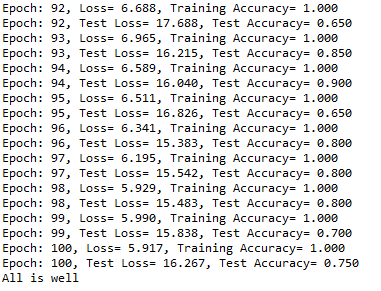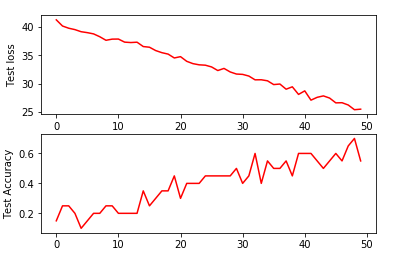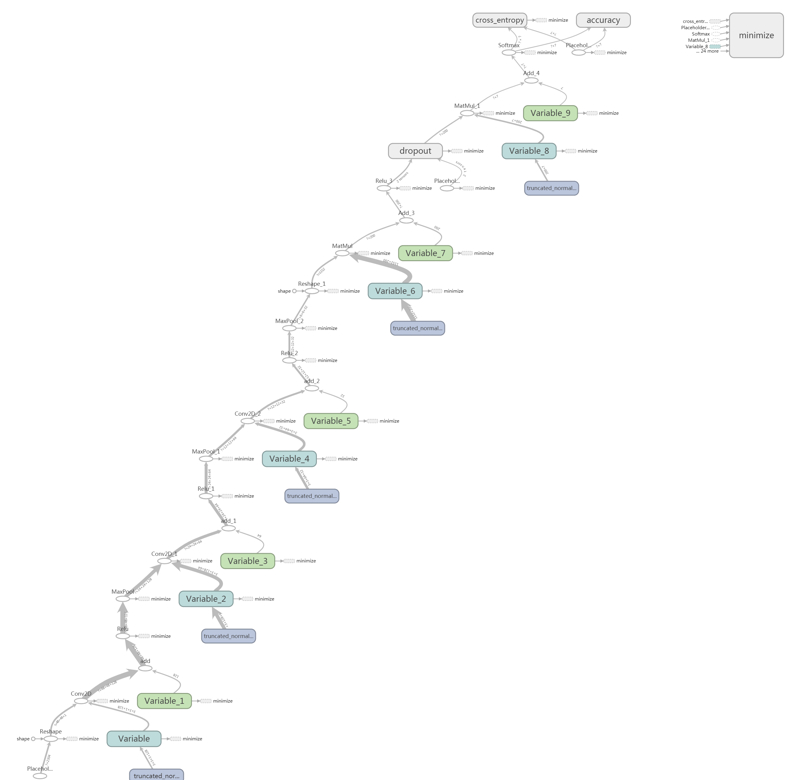# 新手速成---TensorFlow实现jaffe表情识别--step2补充+step3+step4

[48×48]⇀conv2d［3×3］×128⇀pooling⇀conv2d[5×5]×64⇀pooling⇀conv2d[3×3]×32⇀pooling⇀fc[6×6×32]×200⇀dropout⇀softmax[200×7]

epoch=50时
Epoch: 45, Loss= 21.501, Training Accuracy= 0.800
Epoch: 45, Test Loss= 27.431, Test Accuracy= 0.550
Epoch: 46, Loss= 20.672, Training Accuracy= 0.800
Epoch: 46, Test Loss= 26.583, Test Accuracy= 0.600
Epoch: 47, Loss= 21.156, Training Accuracy= 0.750
Epoch: 47, Test Loss= 26.609, Test Accuracy= 0.550
Epoch: 48, Loss= 21.344, Training Accuracy= 0.850
Epoch: 48, Test Loss= 26.205, Test Accuracy= 0.650
Epoch: 49, Loss= 19.028, Training Accuracy= 0.850
Epoch: 49, Test Loss= 25.373, Test Accuracy= 0.700
Epoch: 50, Loss= 19.698, Training Accuracy= 0.800
Epoch: 50, Test Loss= 25.467, Test Accuracy= 0.550
All is well

Epoch=100
Learn rate=0.0001## step4 网络模型的查看

1. 启动CMD，cd 到log文件的上一级目录
2. 激活TensorFlow
3. tensorboard --logdir=logs

## 注意！## 总结11-30905
04-213776
08-011155
04-162405
09-26
07-20
03-25191
10-02